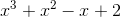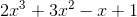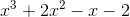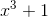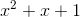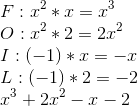## Example Questions

### Example Question #1 : Binomials And Foil

Which of the following expressions is equivalent to: 6x (m2 +yx2 3)?

6xm2 + 6yx3 -18x

6xm2 + 6yx2 -18x

6xm2 + 6yx3 -18

6xm2 + 7x3 -18

xm2 + 7x3 -18

6xm2 + 6yx3 -18x

Explanation:

6x (m2 +yx2 3)= 6x∙m2 + 6xyx2 – 6x∙3= 6xm2 + 6yx3 -18x (Use Distributive Property)

### Example Question #2 : Binomials And Foil

Which of the following expressions is equivalent to: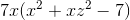?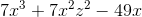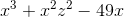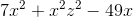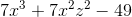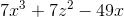Explanation:

Use the distributive property to multiply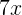by all of the terms in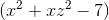: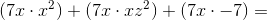### Example Question #3 : Binomials And Foil

Ifandare constants and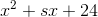is equivalent to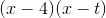, what is the value of?

Cannot be determined from the given information.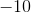Explanation:

The question gives us a quadratic expression and its factored form. From this, we know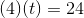At this point, solve for t.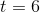Now, we can plug into get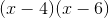.

Now, use FOIL to get s.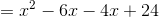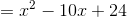### Example Question #4 : Binomials And Foil

Which expression is equal to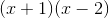?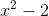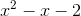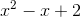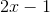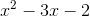Explanation:

In this problem, we are to multiply the two binomials using the FOIL method. This method stands for the order in which you multiply the variables. F stands for the first term of each binomial. O stands for the outside terms, meaning the first term of the first binomial and the last term of the second. I stands for the inside terms, meaning the second term of the first binomial and the first term of the second. L stands for the last term of each binomial. Once you do this, you simply add each part together to recieve your polynomial answer. Here is how our problem is solved: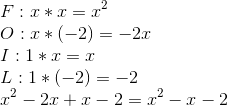### Example Question #5 : Binomials And Foil

Which expression is equal to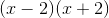?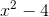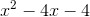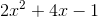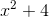Explanation:

In this problem, we are to multiply the two binomials using the FOIL method. This method stands for the order in which you multiply the variables. F stands for the first term of each binomial. O stands for the outside terms, meaning the first term of the first binomial and the last term of the second. I stands for the inside terms, meaning the second term of the first binomial and the first term of the second. L stands for the last term of each binomial. Once you do this, you simply add each part together to recieve your polynomial answer. Here is how our problem is solved: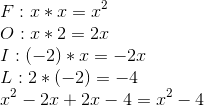### Example Question #6 : Binomials And Foil

Which expression is equal to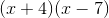?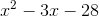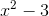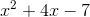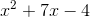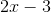Explanation:

In this problem, we are to multiply the two binomials using the FOIL method. This method stands for the order in which you multiply the variables. F stands for the first term of each binomial. O stands for the outside terms, meaning the first term of the first binomial and the last term of the second. I stands for the inside terms, meaning the second term of the first binomial and the first term of the second. L stands for the last term of each binomial. Once you do this, you simply add each part together to recieve your polynomial answer. Here is how our problem is solved: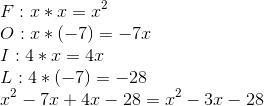### Example Question #7 : Binomials And Foil

Which expression is equal to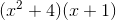?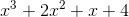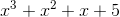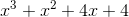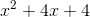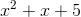Explanation:

In this problem, we are to multiply the two binomials using the FOIL method. This method stands for the order in which you multiply the variables. F stands for the first term of each binomial. O stands for the outside terms, meaning the first term of the first binomial and the last term of the second. I stands for the inside terms, meaning the second term of the first binomial and the first term of the second. L stands for the last term of each binomial. Once you do this, you simply add each part together to recieve your polynomial answer. Here is how our problem is solved: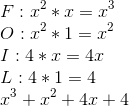### Example Question #8 : Binomials And Foil

Which expression is equal to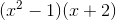?